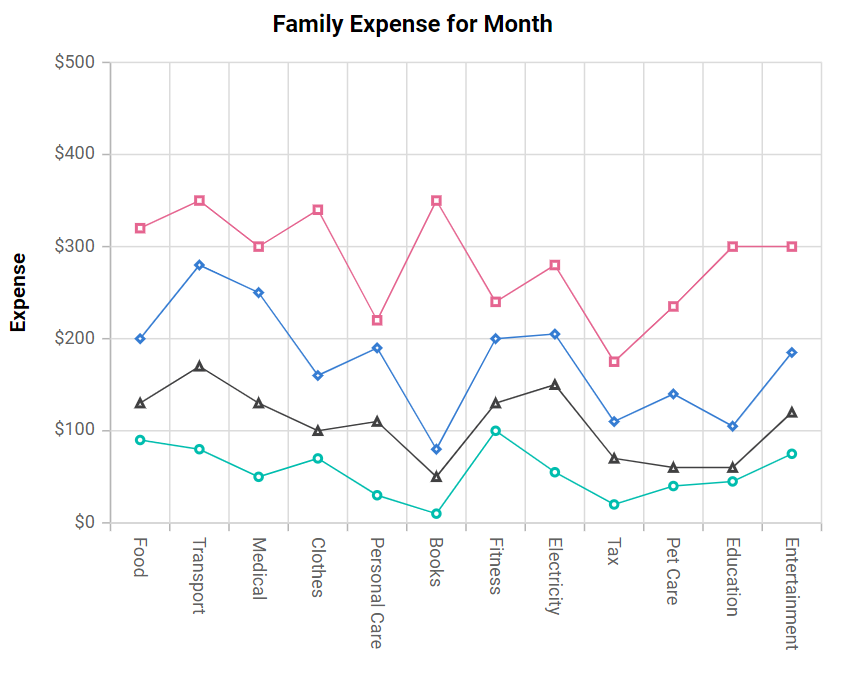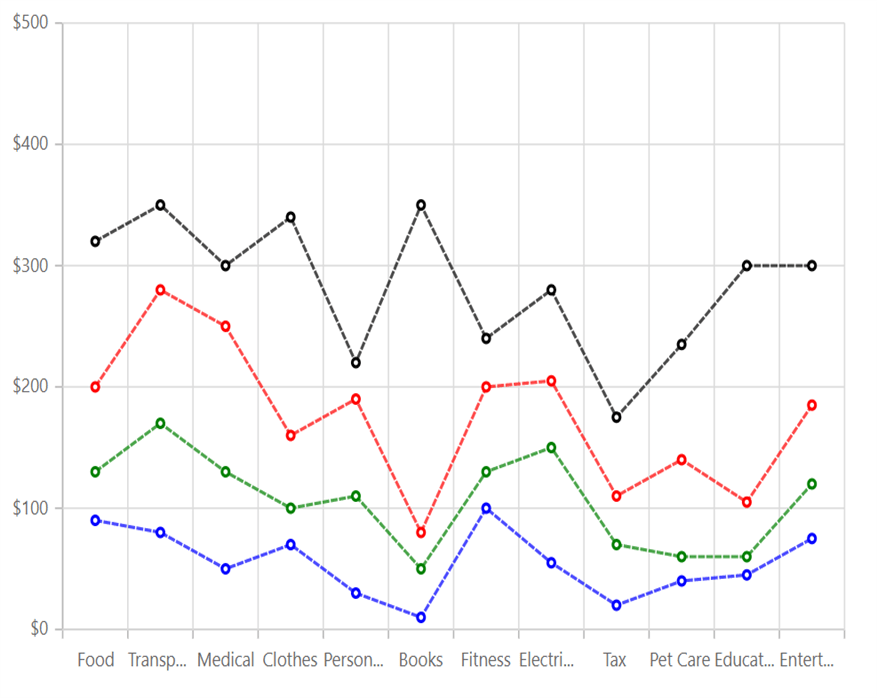# Stacked Line Chart in Blazor Charts Component

9 Dec 20229 minutes to read

## Stacked Line

Stacked Line Chart is a chart with Y values stacked over one another in the series order. It shows the relation between individual values to the total sum of the points. To render a Stacked Line series, set the series Type as StackingLine.

``````@using Syncfusion.Blazor.Charts

<SfChart Title="Family Expense for Month">
<ChartPrimaryXAxis ValueType="Syncfusion.Blazor.Charts.ValueType.Category" Interval="1">
</ChartPrimaryXAxis>

<ChartPrimaryYAxis Title="Expense" Interval="100" LabelFormat="\${value}">
</ChartPrimaryYAxis>

<ChartArea>
<ChartAreaBorder Width="0"></ChartAreaBorder>
</ChartArea>

<ChartSeriesCollection>
<ChartSeries XName="X" DataSource="@ExpenseReports"
YName="Y" Type="ChartSeriesType.StackingLine">
<ChartMarker Visible="true">
</ChartMarker>
</ChartSeries>
<ChartSeries XName="X" DataSource="@ExpenseReports"
YName="Y1" Type="ChartSeriesType.StackingLine">
<ChartMarker Visible="true">
</ChartMarker>
</ChartSeries>
<ChartSeries XName="X" DataSource="@ExpenseReports"
YName="Y2" Type="ChartSeriesType.StackingLine">
<ChartMarker Visible="true">
</ChartMarker>
</ChartSeries>
<ChartSeries XName="X" DataSource="@ExpenseReports"
YName="Y3" Type="ChartSeriesType.StackingLine">
<ChartMarker Visible="true">
</ChartMarker>
</ChartSeries>
</ChartSeriesCollection>
</SfChart>

@code{
public class ChartData
{
public string X { get; set; }
public double Y { get; set; }
public double Y1 { get; set; }
public double Y2 { get; set; }
public double Y3 { get; set; }
}

public List<ChartData> ExpenseReports = new List<ChartData>
{
new ChartData { X = "Food" , Y = 90, Y1 = 40 , Y2= 70, Y3= 120},
new ChartData { X = "Transport", Y = 80, Y1 = 90, Y2= 110, Y3= 70 },
new ChartData { X = "Medical",Y = 50, Y1 = 80, Y2= 120, Y3= 50 },
new ChartData { X = "Clothes",Y = 70, Y1 = 30, Y2= 60, Y3= 180 },
new ChartData { X = "Personal Care", Y = 30, Y1 = 80, Y2= 80, Y3= 30 },
new ChartData { X = "Books", Y = 10, Y1 = 40, Y2= 30, Y3= 270},
new ChartData { X = "Fitness",Y = 100, Y1 = 30, Y2= 70, Y3= 40 },
new ChartData { X = "Electricity", Y = 55, Y1 = 95, Y2= 55, Y3= 75},
new ChartData { X = "Tax", Y = 20, Y1 = 50, Y2= 40, Y3= 65 },
new ChartData { X = "Pet Care", Y = 40, Y1 = 20, Y2= 80, Y3= 95 },
new ChartData { X = "Education", Y = 45, Y1 = 15, Y2= 45, Y3= 195 },
new ChartData { X = "Entertainment", Y = 75, Y1 = 45, Y2= 65, Y3= 115 }
};
}``````NOTE

Refer to our Blazor Stacked Line Chart feature tour page to know about its other groundbreaking feature representations. Explore our Blazor Stacked Line Chart Example to know how to render and configure the Stacked Line type chart.

## Series customization

The following properties can be used to customize the Stacked Line series.

• Fill – Specifies the color of the series.
• Opacity – Specifies the opacity of Fill.
• Width – Specifies the width of the line stroke.
• DashArray – Specifies the dashes of line stroke.
``````@using Syncfusion.Blazor.Charts

<SfChart Title="Family Expense for Month">
<ChartPrimaryXAxis ValueType="Syncfusion.Blazor.Charts.ValueType.Category" Interval="1">
</ChartPrimaryXAxis>

<ChartPrimaryYAxis Title="Expense" Interval="100" LabelFormat="\${value}">
</ChartPrimaryYAxis>

<ChartArea>
<ChartAreaBorder Width="0"></ChartAreaBorder>
</ChartArea>

<ChartSeriesCollection>
<ChartSeries XName="X" Width="2" DashArray="5,1" DataSource="@ExpenseReports"
YName="Y" Fill="blue" Opacity="0.7" Type="ChartSeriesType.StackingLine">
<ChartMarker Visible="true">
</ChartMarker>
</ChartSeries>
<ChartSeries XName="X" Width="2" DashArray="5,1" DataSource="@ExpenseReports"
YName="Y1" Fill="green" Opacity="0.7" Type="ChartSeriesType.StackingLine">
<ChartMarker Visible="true">
</ChartMarker>
</ChartSeries>
<ChartSeries XName="X" Width="2" DashArray="5,1" DataSource="@ExpenseReports"
YName="Y2" Fill="red" Opacity="0.7" Type="ChartSeriesType.StackingLine">
<ChartMarker Visible="true">
</ChartMarker>
</ChartSeries>
<ChartSeries XName="X" Width="2" DashArray="5,1" DataSource="@ExpenseReports"
YName="Y3" Fill="black" Opacity="0.7" Type="ChartSeriesType.StackingLine">
<ChartMarker Visible="true">
</ChartMarker>
</ChartSeries>
</ChartSeriesCollection>
</SfChart>

@code{
public class ChartData
{
public string X { get; set; }
public double Y { get; set; }
public double Y1 { get; set; }
public double Y2 { get; set; }
public double Y3 { get; set; }
}

public List<ChartData> ExpenseReports = new List<ChartData>
{
new ChartData { X = "Food" , Y = 90, Y1 = 40 , Y2= 70, Y3= 120},
new ChartData { X = "Transport", Y = 80, Y1 = 90, Y2= 110, Y3= 70 },
new ChartData { X = "Medical",Y = 50, Y1 = 80, Y2= 120, Y3= 50 },
new ChartData { X = "Clothes",Y = 70, Y1 = 30, Y2= 60, Y3= 180 },
new ChartData { X = "Personal Care", Y = 30, Y1 = 80, Y2= 80, Y3= 30 },
new ChartData { X = "Books", Y = 10, Y1 = 40, Y2= 30, Y3= 270},
new ChartData { X = "Fitness",Y = 100, Y1 = 30, Y2= 70, Y3= 40 },
new ChartData { X = "Electricity", Y = 55, Y1 = 95, Y2= 55, Y3= 75},
new ChartData { X = "Tax", Y = 20, Y1 = 50, Y2= 40, Y3= 65 },
new ChartData { X = "Pet Care", Y = 40, Y1 = 20, Y2= 80, Y3= 95 },
new ChartData { X = "Education", Y = 45, Y1 = 15, Y2= 45, Y3= 195 },
new ChartData { X = "Entertainment", Y = 75, Y1 = 45, Y2= 65, Y3= 115 }
};
}``````NOTE

Refer to our Blazor Charts feature tour page for its groundbreaking feature representations and also explore our Blazor Chart Example to know various chart types and how to represent time-dependent data, showing trends at equal intervals.